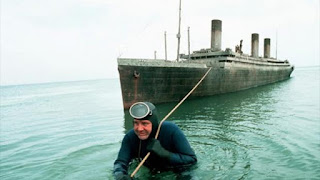# Important Boats and Streams Questions for IBPS PO Pre Exam 2016### Important Points :

If the speed of the a boat in still water is X kmph and speed of the stream is Y kmph
• Speed of Down Stream (DS) = (X+Y) kmph
• Speed of Up Stream (US) = (X - Y ) kmph
• Speed of a boat in still water = 1/2 ( US + DS )
• Speed of Steam  = 1/2 ( US - DS )

#### Ques 1.

Pavan  can row with a speed of 7kmph in still water . What will be his speed with stream , If the speed of stream is 3 kmph ?

Solution :

Speed with stream = 7+3 = 10 kmph

#### Ques 2.

What time will be taken by a boat to cover a distance of 80 km along the stream , if speed of boat in still water is 6 kmph and speed of stream is 2 kmph ?

Solution :

Along with stream = Down Stream  = 6+2 = 8 kmph

Time taken = 80/8 = 10 kmph

#### Ques 3.

A boat takes 4 hours to 56 km downstream and 7 hours to row the same distance upstream . Find the boat's rate in still water and rate of current ?

Solution :

Speed of Downstream = 56/4 = 14 kmph
Speed of Upstream = 56/7 = 8 kmph

Speed of boat in still water = (14+8)/2 = 11 kmph
Speed of Current = (14-8)/2 = 3 kmph

#### Ques 4.

A man can row 10 kmph in still water . It takes him twice as long to row up as to row down the river . Find the rate of stream ?

Solution :

Man speed = 10 kmph
Stream speed = X kmph

==> Time taken to travel downstream = 2 * Time taken to travel upstream
==> 10 + X = 2*(10-X)
==> X = 30/3 kmph

#### Ques 5.

Ravi can row a certain distance downstream in 8 hours  and can return the same distance in 12 hours . If the stream flows at the rate of 4 kmph , then find the speed of Ravi in still water ?

Solution :

==>. 8(x+4) = 12(x-4)
==> 8x + 32 = 12x - 48
==> X = 80/4 = 20 kmph

#### Ques 6.

A man speed in still water is 15 kmph , while river is flowing with a speed of 3 kmph and time taken to cover a certain distance upstream is 3 hours more than taken to cover the same distance downstream . Find the distance .

Solution :

Speed of Downstream = 15+3 = 18 kmph
Speed of Upstream = 15-3 = 12 kmph

==> X/12  -  X/18 = 3
==> X = 36*3
==>  X = 108 km

#### Ques 7.

Raghu rows in still water with a speed of 4.5 kmph to go to a certain place and to come back . Find his average speed for the whole journey , if the river is flowing with a speed of 1.5 kmph ?

Solution :

Raghu's speed upstream = 4.5 - 1.5 = 3 kmph
Ragu's speed downstream = 4.5+1.5 = 6 kmph

Distance = X km

Time Taken in upstream = X/3
Time Taken in downstream = X/6

Average Speed = 2X/(X/3 + X/6 ) = 4 kmph#### What's trending in BankExamsToday

Smart Prep Kit for Banking Exams by Ramandeep Singh - Download here